In this write-up, we'll go over the solution for the medium difficulty crypto challenge MOVs Like Jagger that requires the exploitation of a vulnerable elliptic curve.

## Challenge Description 📄

While following the breadcrumbs from the secret messages you managed to recover from Broider, Bonnie gathered more information from his well trusted "Tsirakia" and found out that the messages were about the destinations of a STS (Space Transportation Ship). The STS, named "Paketo", is a notoriously large spaceship that transports military supplies for the Golden Fang mercenary army. After spending some time observing the seemingly random trajectory of Paketo, you and Paulie manually plotted the delivery locations and realized that the STS's orbit forms an elliptic curve. Your spaceship's advanced technology GPS has calculated the exact parameters of the curve. Can you somehow predict Paketo's final destination and hijack it?

## The application at-a-glance 🔍

If you visit the homepage of the application, you will see a GPS like system indicating the movement of the STS called "Paketo". The only information available to us is the current coordinates and the coordinates from which the ship started.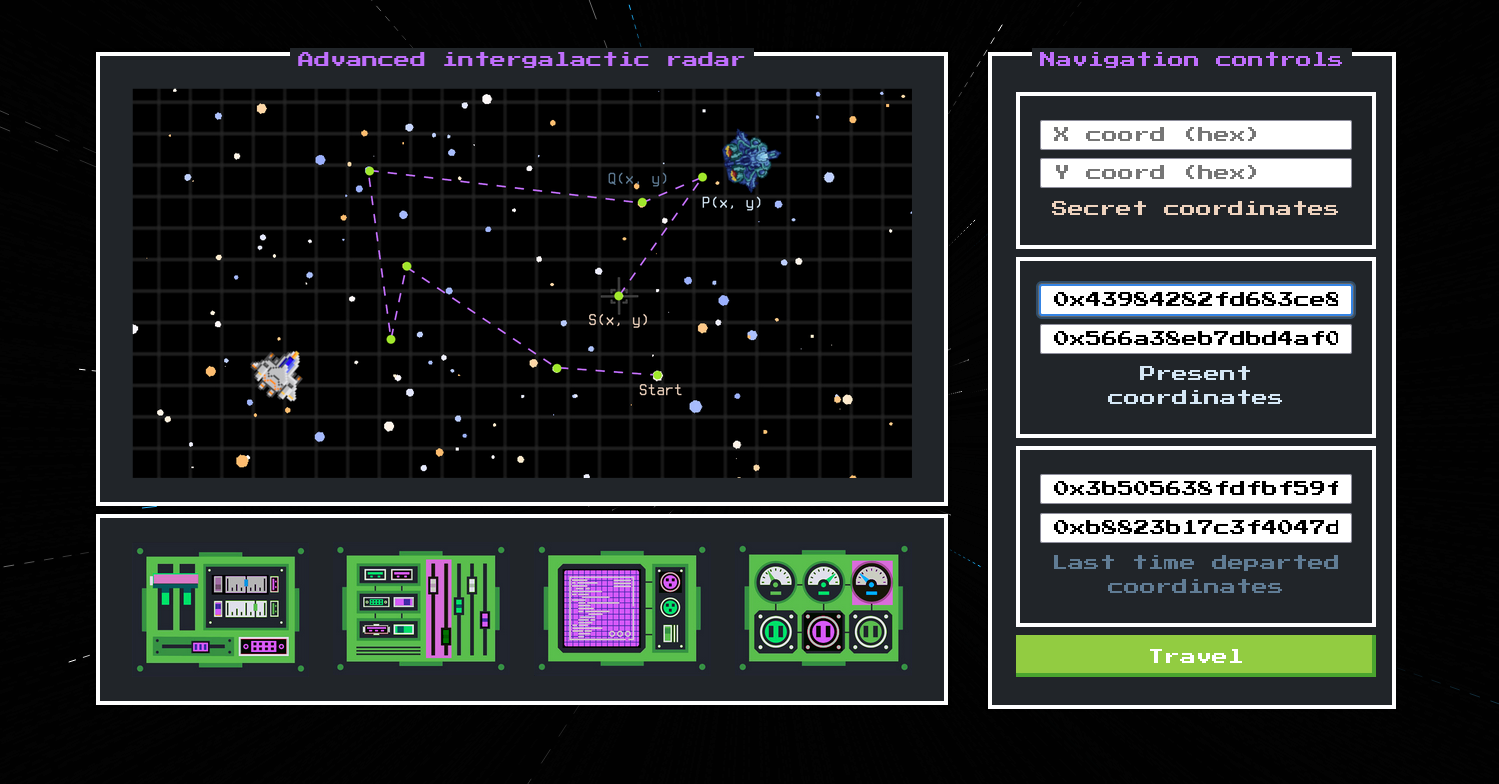We can try entering random data into the secret coordinates field and see what happens when we press the travel button.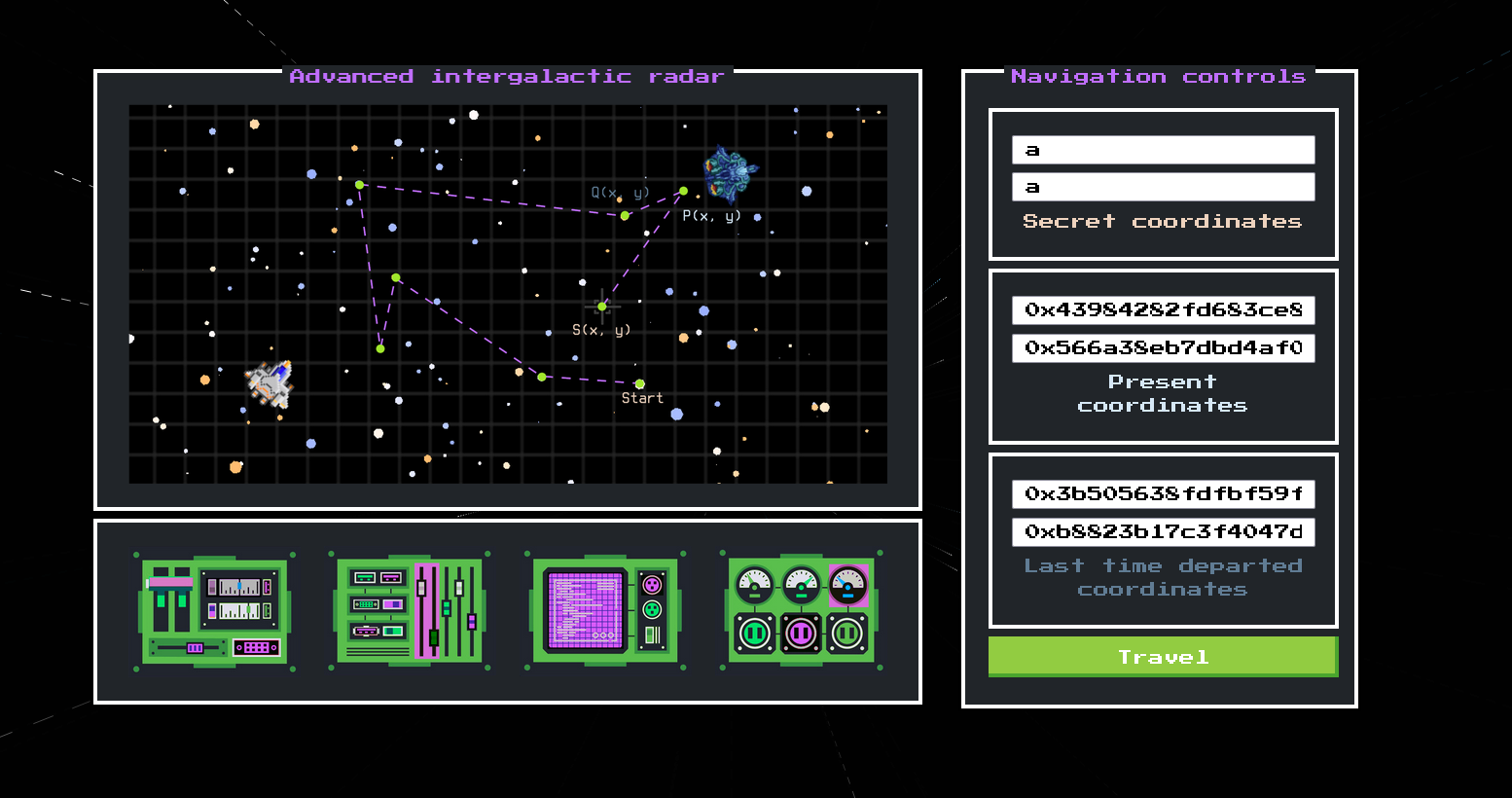We see that we are redirected to a conversation between Klaus and Bonnie, in which Bonnie tells us that we have travelled to Longhir.At this point, we need to start looking at the source code to understand how things work.

## Analysing the source code 📖

There are 3 files available app.py, navigation.py, utils.py

The utils.py script, generates random locations if we enter the wrong coordinates, so we ignore it.

### app.py

We can see that in app.py there is an API provided:

``````@app.route('/api/coordinates', methods=['GET'])
def coordinates():
points = {
'departed_x': hex(Q.x()), 'departed_y': hex(Q.y()),
'present_x': hex(P.x()), 'present_y': hex(P.y())
}
return points

@app.route('/api/get_flag', methods=['POST'])
def get_flag():
try:
travel_result = checkDestinationPoint(request.json, P, nQ, E)
location = generateLocation(travel_result)

if travel_result:
return {"location": location, "flag": FLAG}
else:
return {"location": location}
except ValueError as error:
return {"error": error}

if __name__ == '__main__':
Q, nQ, P, nP = calculatePointsInSpace()

We see that in order to get the flag, we must somehow set `travel_result = True`. The function that sets `travel_result` is called `checkDestinationPoint()` and is imported from navigation.py

The basic workflow of the script is:

1. Initialise and define the parameters for the curve.

2. Compute 2 key pairs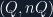(the departed point) and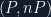(the present point).

3. Check if the coordinates given by the player are valid.

4. The secret point (destination point) is generated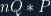and compared with the point we have given. If they match, we get the flag.

Step 1 is translated into code:

``````from ecdsa import ellipticcurve as ecc
from random import randint

a = -35
b = 98
p = 434252269029337012720086440207
Gx = 16378704336066569231287640165
Gy = 377857010369614774097663166640
ec_order = 434252269029337012720086440208

E = ecc.CurveFp(p, a, b)
G = ecc.Point(E, Gx, Gy, ec_order)``````

Step 2 is implemented with the `calculatePointsInSpace()` and `generateKeyPair()`:

``````def generateKeyPair():
private = randint(1, 2**64)
public = G * private
return(public, private)

def calculatePointsInSpace():
Q, nQ = generateKeyPair()
P, nP = generateKeyPair()
return [Q, nQ, P, nP]``````

Step 3 is implemented with the `checkCoordintates()` function. This is just a simple method to see if the points entered by the player are valid.

Step 4 is the win condition. If the `secret_point` and the point we entered match, we get the flag

``````    secret_point = P * nQ
same_x = destination_point.x() == secret_point.x()
same_y = destination_point.y() == secret_point.y()

if (same_x and same_y):
return True
else:
return False``````

To summarise once again:
To get the flag, we need to make `checkDestinationPoint()` return True. To do this, we must somehow predict what the secret locationis.
Let’s search for the vulnerability now

## Searching for the bugs 👾

One thing that should strike us when we look at the challenge is that the curve appears to use custom parameters. Let us examine these.

``````a = -35
b = 98
p = 434252269029337012720086440207``````

There are a lot of vulnerabilities we can check in custom elliptic curves, but really the title of the challenge is a big clue to the attack. If we search for something along the lines of "MOV attack elliptic curves" we will find a lot of resources.

## Understanding the vulnerability 🔐

In ECC it is difficult to solve the discrete logarithm problem.

The basic idea of this attack is that the discrete logarithm problem can be moved from an elliptic curve defined over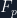(finite field) to the multiplicative group of the finite field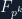when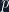is divided by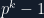. If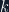is small enough (k < 6), the discrete log becomes easier to calculate than on the curve. Let us first check thatis small:

``````from sage.all import *

a = -35
b = 98
p = 434252269029337012720086440207
Gx = 16378704336066569231287640165
Gy = 377857010369614774097663166640

E = EllipticCurve(GF(p), [a, b])

k = 1
while (p**k - 1) % E.order():
k += 1

print(k)``````

In our case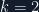everything seems to be set.

``````┏━[/tmp]
┗━━ ■ python3 check_k.py
2``````

## Exploitation 🔓

### MOV attack

Analysing the source code in the above section, we concluded in step 5 that we must somehow find.is known, but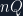is not.

We know  that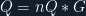, but in ECC it is really hard to solve this problem. If only we had a vulnerability... Oh, wait, we do! The MOV attack. Let us implement it.

A good abstraction of the attack algorithm can be found here.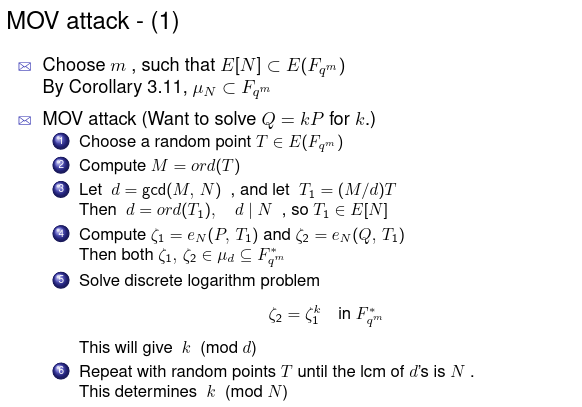Also a write-up that can help a lot of users with the implementation is this one.

The problem is solved for,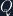, whereand `nQ = randomint(1, 2**64)`

We will start by working in``Ee = EllipticCurve(GF(p**k, 'y'), [a, b])``

We choose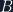such thatandare linearly independent.

``R = Ee.random_point() m = R.order() d = gcd(m, G.order()) B = (m // d) * R ``

After that we take the Weil pairing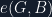and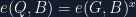``````Ge = Ee(G)
Qe = Ee(Q)
n = G.order()
alpha = Ge.weil_pairing(B, n)
beta = Qe.weil_pairing(B, n)``````

Finally we compute the discrete logarithm in this finite field to find the secret multiplier.

``nQ = beta.log(alpha) ``

The complete function is:

``````def movAttack(G, Q):
k = 1
while (p**k - 1) % E.order():
k += 1

Ee = EllipticCurve(GF(p**k, 'y'), [a, b])

R = Ee.random_point()
m = R.order()
d = gcd(m, G.order())
B = (m // d) * R

assert G.order() / B.order() in ZZ
assert G.order() == B.order()

Ge = Ee(G)
Qe = Ee(Q)

n = G.order()
alpha = Ge.weil_pairing(B, n)
beta = Qe.weil_pairing(B, n)

print('Computing log...')
nQ = beta.log(alpha)
return nQ``````

There are 2 ways to get the points and calculate the secret coordinates. We can use the UI or the API. I will provide the manual way for aesthetic reasons and then the automatic solver that uses the API.

### Manual exploitation

Let’s start by defining the curve:

``````from sage.all import *

a = -35
b = 98
p = 434252269029337012720086440207
Gx = 16378704336066569231287640165
Gy = 377857010369614774097663166640
E = EllipticCurve(GF(p), [a, b])``````

Let’s visit the website again.